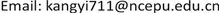1. 引言

2. 数学模型2.1. 重构方法数学描述

V = A Y (1)

Y ——实际过程参数矩阵(如：流速，体积分数等)，维度为 m × 1 ；

A ——已知变换矩阵，维度为 x × m ；

V ——测量所得的过程参数矩阵，维度为 x × 1 。

Y R = W α (2)

Y R = ∑ i = 1 k α i W i (3)

Y R ——重构过程参数矩阵，维度为 m × 1 ；

W ——基矩阵，维度为 m × k ；

α ——重构系数矩阵，维度为 k × 1 ( k ≪ m )；

α i ——第 i t h 节点基矩阵线性组合系数。

Z m × n = W m × k H k × n + D m × n (4)

Z ——流场过程参数样本矩阵(包含n种不同工况)，维度为 m × n ；

W ——基矩阵，维度为 m × k ；

H ——分解权重矩阵，维度为 k × n ；

D ——分解误差矩阵，维度为 m × n 。

V ≈ A W α (5)

e R = ‖ Y - Y R ‖ 1 ‖ Y R ‖ 1 (7)

2.2. 非负矩阵分解(NMF)

Z m × n = W m × k H k × n + D m × n (8)

Z m × n ≈ W m × k H k × n = Z ^ m × n (9)

where W m × k ≥ 0 ， H k × n ≥ 0 ；

‖ B − C ‖ 2 = ∑ i , j ( B i , j − C i , j ) 2 (10)

Kullback-Leibler散度法：

F ( B ‖ C ) = ∑ i , j ( B i , j log B i , j C i , j − B i , j + C i , j ) ≥ 0 ，(当且仅当 B = C ； F = 0 ) (12)

min ‖ Z − W H ‖ 2 ,   s . t . W ≥ 0 , H ≥ 0 (11)

min F ( Z ‖ W H ) ,   s . t . W ≥ 0 , H ≥ 0 (12)

3. CFD模型

4. 重构结果

4.1. 体积分数重构结果

4.2. 速度重构结果

5. 讨论

5.1. 基矩阵

5.2. 采样率

5.3. 泄露

6. 结论

1. 基于有限的过程参数测量数据，所提出的方法可用于重构复杂机械结构周围的流场。不同泄露情况下，都可以较为准确地重构流场，尤其是对于类似流场速度这类不存在较大数量级差异的过程参数，可以精确地重构流场。

2. 相对重构误差主要受基矩阵、采样率以及过程参数数量级的影响。在选取合适的基矩阵的前提下，采样率越低重构误差越大并且过程参数数量级的差异是误差的主要来源。

3. 在实际情况中，所提出的重构方法避免了多相流检测过程中复杂敏感场的计算，而且相较于CFD模拟方法和插值方法，重构所需的时间大幅缩减。